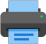Lista de exercícios do ensino médio para impressãoSelect all.
print selected exercises
AB is the diameter of the circle whose center is O and the triangle ABC is inscribed in. The quotient $\,\dfrac{s}{S}\,$ where the area $\,s\,$ of the triangle ACO is divided by the area $\,S\,$ of the triangle COB is:
a)
$\,\dfrac{5}{4}\,$
b)
$\,\dfrac{4}{3}\,$
c)
$\,\dfrac{3}{4}\,$
d)
$\,1\,$
e)
$\,\dfrac{\sqrt{3}}{2}\,$